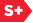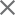### Proceedings Paper

Numerical approximation of scalar diffraction through first order optical systems
Format Member Price Non-Member Price
PDF \$17.00 \$21.00

Paper Abstract

Luneburg's first order optical systems consist of sections of free space, lenses, and all possible combinations of these. The linear canonical transform (LCT), a parameterised linear integral transform, may be used to model the paraxial propagation of scalar optical fields through such systems. We consider the propagation of quasi-monochromatic, coherent wave fields, though more general calculations are possible. Numerical approximation of such systems is an active area of research, of interest for system design and analysis. We consider methods for the determination of the sampling requirements for the wave fields at the input and output of such calculations, in conjunction with the discretisation of the transform. We illustrate these considerations using phase space diagrams (PSDs), making use of the LCT's simple co-ordinate transforming effect on such diagrams. We discuss the implications of the cross-terms present in the Wigner distribution function, which are ignored in such PSD-based analyses, for the accuracy of the simulations and for the selection of sampling schemes. We examine the available algorithms for performing the transformations in O(N log N) time. In particular, we consider the relative merits of algorithms which decompose the optical system into special cases for which fast algorithms are better developed and also algorithms which decompose the calculations into smaller ones iteratively.

Paper Details

Date Published: 14 May 2010
PDF: 10 pages
Proc. SPIE 7717, Optical Modelling and Design, 77171A (14 May 2010); doi: 10.1117/12.854053
Show Author Affiliations
John J. Healy, Univ. College Dublin (Ireland)
John T. Sheridan, Univ. College Dublin (Ireland)

Published in SPIE Proceedings Vol. 7717:
Optical Modelling and Design
Frank Wyrowski; John T. Sheridan; Jani Tervo; Youri Meuret, Editor(s)